# How to obtain ANOVA Single factor in Excel

In this Example, we will learn how to perform single factor ANOVA in Excel 2016.

A single factor ANOVA is used to test the null hypothesis. Which means the mean from all the population are all equal and alternate hypothesis is at least one among the mean is different.

All of the above might be confusing for some people, so let’s gear up & start learning ANOVA single factor in excel with the example.

Here we have marks of Three students in the table.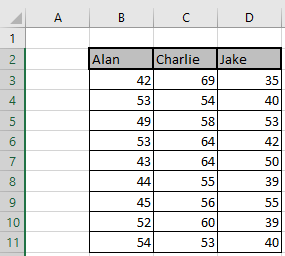Select the tab Data > Data Analysis as shown in below screenshot.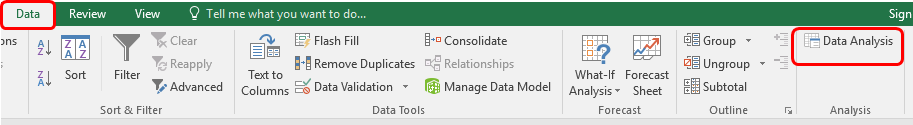Click on Data Analysis and its dialog box appears.
Now select Anova: Single factor and click OK.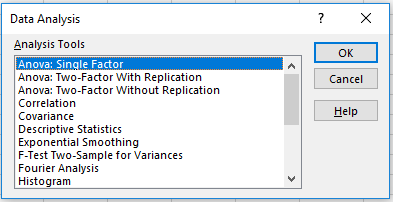Anova: Single factor dialog box appears. Fill the options as shown below.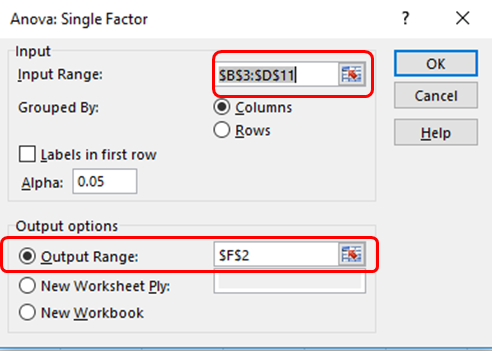Click OK to get the stats for your data.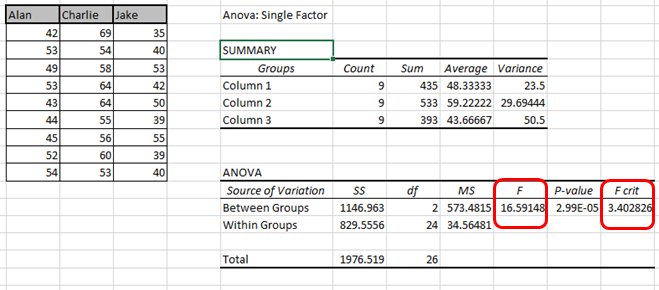Conclusion: if the value of F > F crit, we reject the null hypothesis. In this case, 16.59 > 3.40. Therefore, we reject the null hypothesis. The means of the three populations are not all equal. At least one of the means is different.

Hope you got how to test ANOVA single factor tool in Excel 2016. Find more articles on Data Analysis here. Please state your query in the comment box below. We will help you.

Popular Articles:

50 Excel Shortcuts to Increase Your Productivity

How to use the VLOOKUP Function in Excel

How to use the COUNTIF function in Excel

How to use the SUMIF Function in Excel

1.